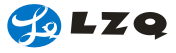Illustration method of geometric tolerance
 ◆.Illustration method of geometric tolerance
 Type of tolerance Mark Definition of tolerance zone Note to illustration Form tolerance Straightness When there is symbol φ in front of the numerical value that expresses tolerance zone, it means this tolerance zone, the area in the cylinder with diameter of t If the tolerance frame is after the diameter of the cylinder, it means the axial line of the cylinder could have 0.08mm tolerance Flatness Tolerance zone: area enclosed by two average planes with a distance of t This surface must be between two parallel planes with a distance of t Roundness Object plane's tolerance zone is area which two concentric circles with a distance of t The circumference of right-angle section of any axis must be between two concentric circles with a distance of t in the same plane Cylindricity Tolerance zone is area in which two coaxial cylinder plane with a distance of t Object plane must be between two coaxial cylinder surfaces with a distance of 0.1mm Line profile Tolerance zone is area enclosed by two profile lines of a circle with a diameter of t when the center is in the theoretically correct contour line The contour of the object in any section that is parallel with projective surface must be between two profile lines of a circle with a diameter of 0.04mm when the center is in the theoretically correct contour plane. Surface profile Tolerance zone is area enclosed by two profile planes of a sphere with a diameter of t when the center is in the theoretically correct contour line Object plane must be between two profile planes of a sphere with a diameter of 0.02mm when the center is in the theoretically correct contour plane Orientation tolerance Parallelism When there is symbol φ in front of the numerical value that expresses tolerance zone, this tolerance zone is area in the cylinder with a diameter of t that is perpendicular with the datum plane Indication surface of indicating line arrow must be parallel with datum surface, and be between two planes with a distance of 0.01mm in the direction of indicating line arrow Perpendicularity When there is symbolφ in front of the numerical value that expresses tolerance zone ,this tolerance zone is area in the cylinder width a diameter of that is perpendicular width the datum plane. The cylinder axis indicated by indicating line arrow must be perpendicular with datum surface and in the cylinder with a diameter of 0.01mm. Angularity Tolerance zone is area enclosed by two parallel planes with a distance of tand parallel width datum plane. The surface indicated by indicating line arrow, must be theoretically 40° inclined from datum plane A, and be between two parallel planes with a distance of 0.08 in the direction of indicating line arrow. Tolerance in position Position Tolerance zone is the area in a circle or sphere with a diameter of t with theoretically correct position (hereinafter referred to as "positive position" as the center. The point indicated by indicating line arrow, must be in the circle with a diameter of 0.03mm with the positive position that is 60mm from datum straight line A and 100mm from datum straight line B as the center. Concentricity When there is symbol φ in front of the numerical value that expresses tolerance zone, this tolerance zone is area in the cylinder with a diameter of that is parallel with the datum axis line The axis indicated by indicating line arrow, must be in the cylinder with a diameter of 0.01mm with datum axial straight line A as axis Symmetry Tolerance zone is the area symmetry with the datum plane, and enclosed by two parallel planes with a distance of "t". The center plane indicated by indicating line arrow must be between two parallel planes with a distance of 0.08mm and symmetric with datum center plane A. Vibration tolerance Circular runout Measure implementation surface (measure sur face) Tolerance surface attached Tolerance zone is area between two concentric circles with a distance of t in the direction of radius and has the same center with datum axial straight line in any measured plane perpendicular with datum axial straight line The vibration in the direction of cylinder plane radius indicated by indicating line arrow is in any measured plane perpendicular with datum axialstraight line when datum straight line A-B rotates a round and it will not exceed 0.1mm. Total runout Tolerance zone is area between two coaxial cylinders with a distance of t in the direction of radius and has the same axis with datum axial straight line The complete vibration in the direction of cylinder plane radius indicated by indicating line arrow, when in datum straight line A-B's rotating cylindrical part, any point on cylinder surface will not exceed 0.1mm
 The lines used in definition column of tolerance zone have the following meanings Thick real line or dashed : form Thick one-dot dashed: data available Thin real line or dashed: tolerance zone Thin one-dot dashed: center line Thin two-dot dashed: complementary projective surface or section Thick two-dot dashed: projection of profile to complementary projective surface or fracture surface-3-